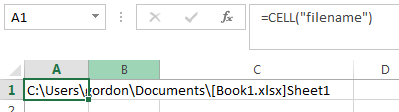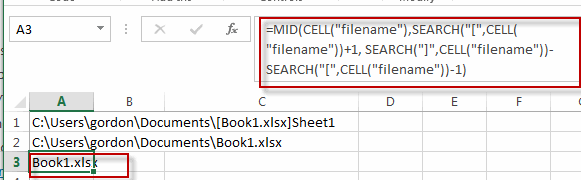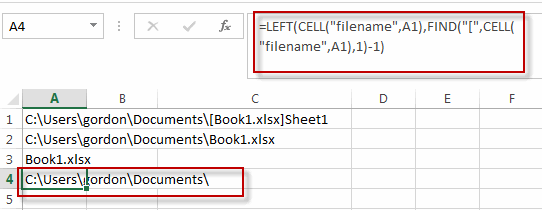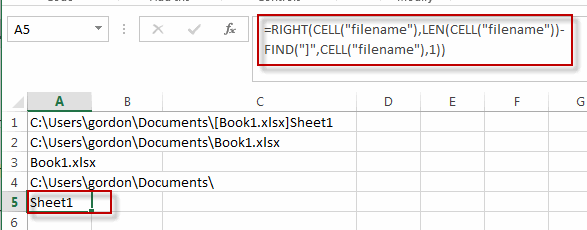# Insert The File Path and Filename into Cell

This post will guide you how to insert the file path and filename into a cell in Excel. Or how to add a file path only in a specified cell with a formula in Excel. Or how to add the current workbook name into a cell in Excel. How do I insert the current worksheet name into a Cell with a formula.

## Insert File Path and Filename into Cell

If you want to insert a file path and filename into a cell in your current worksheet, you can use the CELL function to create a formual. Just like this:

`=CELL("filename")`

Type this formula into a blank cell, and then press Enter key in your keyboard.This fromula will return the entire path, filename, and tab name of the current worksheet.

If you want to strip out the brackets and the sheet name, you can use the following formula based on the SUBSTITUTE function, the LEFT function, the CELL function and the FIND function:

`=SUBSTITUTE(LEFT(CELL("filename"),FIND("]",CELL("filename"))-1),"[","")`

## Insert Filename Only into Cell

If you only want to insert the workbook name into a cell, you can use a formula based on the MID function, the SEARCH function, and the CELL function. Just like this:

`=MID(CELL("filename"),SEARCH("[",CELL("filename"))+1, SEARCH("]",CELL("filename"))-SEARCH("[",CELL("filename"))-1)`

Type this formula into a blank cell and then press Enter key.You will see this formula will return only the current workbook name in Cell.

## Insert Filepath Only into Cell

If you just want to insert the File Path into a cell, you can create a formula based on the LEFT function, the Cell function,and the FIND function. Just like this:

`=LEFT(CELL("filename",A1),FIND("[",CELL("filename",A1),1)-1)`

Type this formula into a blank cell and then press Enter key to apply this formula.This formula will return only the File path of the current workbook.

## Insert Worksheet Name into Cell

If you want to get the worksheet name only and then insert it into one cell, you can create a formula based on the RIGHT function, the LEN function, the CELL function and the FIND function. Just like this:

`=RIGHT(CELL("filename"),LEN(CELL("filename"))-FIND("]",CELL("filename"),1))`

Type this formula into a blank cell and then press Enter key to apply this formula.This formula will return only the current worksheet name in a cell.

### Related Functions

• Excel Find function
The Excel FIND function returns the position of the first text string (substring) from the first character of the second text string.The FIND function is a build-in function in Microsoft Excel and it is categorized as a Text Function.The syntax of the FIND function is as below:= FIND  (find_text, within_text,[start_num])…
• Excel MID function
The Excel MID function returns a substring from a text string at the position that you specify.The syntax of the MID function is as below:= MID (text, start_num, num_chars)…
• Excel CELL function
The Excel CELL function returns information about the formatting, location, size, or contents of a cell.The syntax of the CELL function is as below:= CELL (info_type,[reference])…
• Excel Substitute function
The Excel SUBSTITUTE function replaces a new text string for an old text string in a text string.The syntax of the SUBSTITUTE function is as below:= SUBSTITUTE  (text, old_text, new_text,[instance_num]) ….
• Excel LEFT function
The Excel LEFT function returns a substring (a specified number of the characters) from a text string, starting from the leftmost character.The LEFT function is a build-in function in Microsoft Excel and it is categorized as a Text Function.The syntax of the LEFT function is as below:= LEFT(text,[num_chars])…
• Excel SEARCH function
The Excel SEARCH function returns the number of the starting location of a substring in a text string.The syntax of the SEARCH function is as below:= SEARCH  (find_text, within_text,[start_num])…
• Excel LEN function
The Excel LEN function returns the length of a text string (the number of characters in a text string).The LEN function is a build-in function in Microsoft Excel and it is categorized as a Text Function.The syntax of the LEN function is as below:= LEN(text)…
• Excel RIGHT function
The Excel RIGHT function returns a substring (a specified number of the characters) from a text string, starting from the rightmost character.The syntax of the RIGHT function is as below:= RIGHT (text,[num_chars])…

Related Posts

Find and Replace Multiple Values

This post will guide you how to find and replace multiple values at once with VBA macro or using formula  in Excel. How do I make multiple find and replace in Excel. Suppose that you have a few cells containing ...

Extract Last Two Words From Multiple Cells

Just assume that you have a few cells containing values/words and you want to extract the last two words from each cell into another separate cell; then you might think that it's not a big deal; because you would prefer ...

Extract Multiple Lines From A Cell

Suppose that you have listed some text in a single cell which is separated by the line break(you can do it by pressing ALT + ENTER after entering the text), and now you want to extract multiple lines of text ...

How to Remove All Asterisk Characters from Cells in Excel

Sometimes when we opening an excel spreadsheet to check data, we may notice that there are some asterisks exist in texts for security or other reasons. If you feel these special characters are invalid you can remove them from cells. ...

How to Convert Date Format from Dot MM.DD.YYYY to Slash MM/DD/YYYY in Excel

We often enter dates in excel tables, and we can enter current date by quickly press ‘Ctrl+;’ simultaneously. If in some cases we enter a date format that is not what we want, we have to switch the date format ...

How to Remove Quotes for Text or Strings in Excel

When we importing data from other source into Excel we may import quotes or quotations from initial file. And we want to remove these quotes from current file, if we remove them one by one it needs a lot of ...

How to Remove Specific Character from Text Cell in Excel

This post will guide you how to remove a specific character from text cell in Excel. How do I remove certain character from a cell with a formula in Excel 2013/2016. Remove Specific Character Using Find and Replace function Remove ...

How to Count Comma Separated Value in One Cell in Excel

This post will guide you how to count values in a single cell separated by commas with a formula in Excel. How do I count comma separated values in one cell in Excel 2013/2016. Is it possible to have a ...

How to Count the Number of Letters or Numbers separately in a Cell in Excel

This post will guide you how to count the number of a given character in a cell in Excel. How do I count only numbers within a single cell excluding all letters and other characters with a formula in Excel. ...

How to Remove the First/Last Word from Text string in Cell

This post will guide you how to remove the first and the last word from a text string in cells in Excel. How do I use a formula to remove first and last word of a text string in Excel. ...

Sidebar